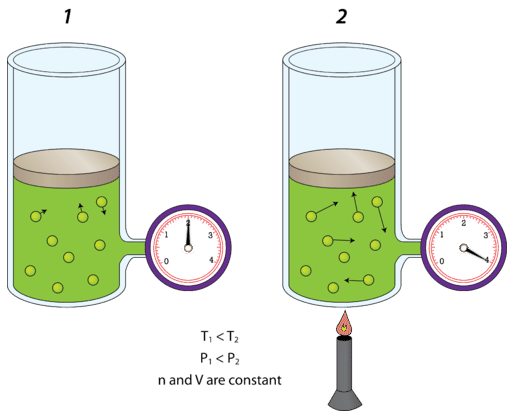# 7.4: Factors Affecting Gas Pressure

$$\newcommand{\vecs}{\overset { \rightharpoonup} {\mathbf{#1}} }$$ $$\newcommand{\vecd}{\overset{-\!-\!\rightharpoonup}{\vphantom{a}\smash {#1}}}$$$$\newcommand{\id}{\mathrm{id}}$$ $$\newcommand{\Span}{\mathrm{span}}$$ $$\newcommand{\kernel}{\mathrm{null}\,}$$ $$\newcommand{\range}{\mathrm{range}\,}$$ $$\newcommand{\RealPart}{\mathrm{Re}}$$ $$\newcommand{\ImaginaryPart}{\mathrm{Im}}$$ $$\newcommand{\Argument}{\mathrm{Arg}}$$ $$\newcommand{\norm}{\| #1 \|}$$ $$\newcommand{\inner}{\langle #1, #2 \rangle}$$ $$\newcommand{\Span}{\mathrm{span}}$$ $$\newcommand{\id}{\mathrm{id}}$$ $$\newcommand{\Span}{\mathrm{span}}$$ $$\newcommand{\kernel}{\mathrm{null}\,}$$ $$\newcommand{\range}{\mathrm{range}\,}$$ $$\newcommand{\RealPart}{\mathrm{Re}}$$ $$\newcommand{\ImaginaryPart}{\mathrm{Im}}$$ $$\newcommand{\Argument}{\mathrm{Arg}}$$ $$\newcommand{\norm}{\| #1 \|}$$ $$\newcommand{\inner}{\langle #1, #2 \rangle}$$ $$\newcommand{\Span}{\mathrm{span}}$$$$\newcommand{\AA}{\unicode[.8,0]{x212B}}$$

The pressure of the air in a basketball has to be adjusted so that the ball bounces to the correct height. Before a game, officials check the ball by dropping it from shoulder height and seeing how far back up it bounces. What would an official do if the ball did not bounce up high enough, or if it bounced too high?

The pressure inside a container is dependent on the amount of gas inside the container. If a basketball does not bounce high enough, an official could remedy the situation by using a hand pump and adding more air to the ball. Conversely, if it bounces too high, the official could let some air out of the ball.

## Factors Affecting Gas Pressure

Recall from the kinetic-molecular theory that gas particles move randomly and in straight lines until they elastically collide with either other gas particles, or with one of the walls of the container. It is these collisions with the walls of the container that define the pressure of the gas. Four variables are used to describe the condition of a gas: pressure $$\left( P \right)$$, volume $$\left( V \right)$$, temperature $$\left( T \right)$$, and the amount of the gas as measured by the number of moles (\left( n \right)\). We will examine separately how the volume, temperature, and amount of gas each affect the pressure of an enclosed gas sample.

### Amount of Gas

The figure below shows what happens when air is added to a rigid container. A rigid container is one that is incapable of expanding or contracting. A steel canister is an example of a rigid container.Figure $$\PageIndex{1}$$: Increase in pressure with increase in number of gas particles. (CC BY-NC; CK-12)

The canister on the left contains a gas at a certain pressure. The attached air pump is then used to double the amount of gas in the canister. Since the canister cannot expand, the increased number of air molecules will strike the inside walls of the canister twice as frequently as they did before. The result is that the pressure inside the canister doubles. As you might imagine, if more and more air is continually added to a rigid container, it may eventually burst. Reducing the number of molecules in a rigid container has the opposite effect, and the pressure decreases.

### Volume

Pressure is also affected by the volume of the container. If the volume of a container is decreased, the gas molecules have less space in which to move around. As a result, they will strike the walls of the container more often, and the pressure increases.

The figure below shows a cylinder of gas whose volume is controlled by an adjustable piston. On the left, the piston is pulled mostly out and the gauge reads a certain pressure. On the right, the piston has been pushed so that the volume of the enclosed portion of the container where the gas is located has been cut in half. The pressure of the gas doubles. Increasing the volume of the container would have the opposite effect, and the pressure of the gas would decrease.Figure $$\PageIndex{2}$$: Decrease in gas volume produced increase in gas pressure. (CC BY-NC; CK-12)

### Temperature

It would be very inadvisable to place a can of soup over a campfire without venting the can. As the can heats up, it may explode. The kinetic-molecular theory explains why. The air inside the rigid can of soup is given more kinetic energy by the heat coming from the campfire. The kinetic energy causes the air molecules to move faster; they impact the container walls more frequently and with more force. The increase in pressure inside may eventually exceed the strength of the can and it will explode. An additional factor is that the soup may begin boiling, which will then aid even more gas and more pressure to the inside of the can.

Shown in the figure below is a cylinder of gas (left) that is at room temperature $$\left( 300 \: \text{K} \right)$$. On the right, the cylinder has been heated until the Kelvin temperature has doubled to $$600 \: \text{K}$$. The kinetic energy of the gas molecules increases, so collisions with the walls of the container are now more forceful than they were before. As a result, the pressure of the gas doubles. Decreasing the temperature would have the opposite effect, and the pressure of an enclosed gas would decrease.Figure $$\PageIndex{3}$$: Increase in temperature produces increase in pressure. (CC BY-NC; CK-12)

## Summary

• An increase in the number of gas molecules, while container volume stays constant, increases pressure.
• A decrease in container volume increases gas pressure.
• An increase in temperature of a gas in a rigid container increases the pressure.

7.4: Factors Affecting Gas Pressure is shared under a CC BY-NC license and was authored, remixed, and/or curated by LibreTexts.